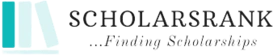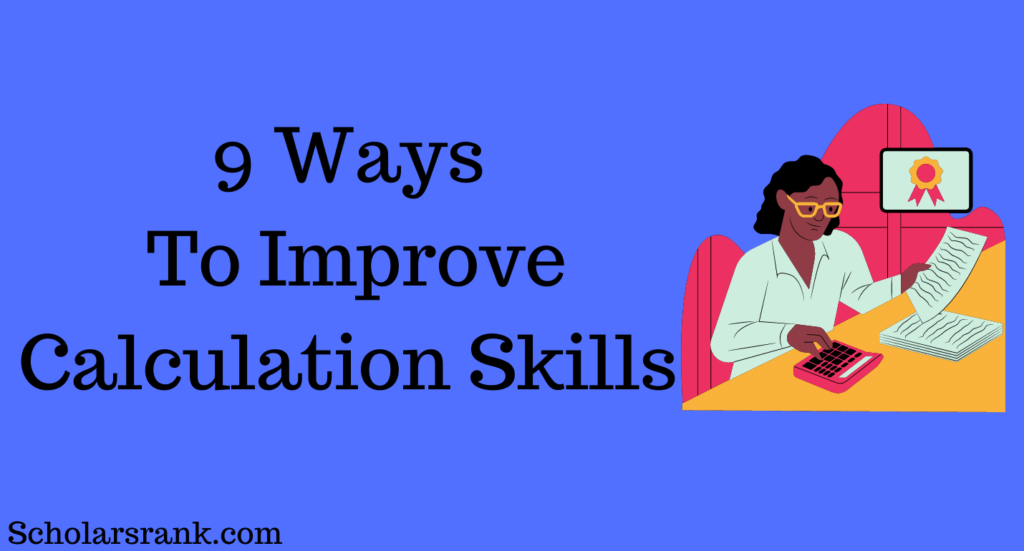# 9 Ways To Improve Calculation Skills

##### Share## Introduction

Understanding how to Improve Calculation Skills is crucial. It is difficult to imagine our world without math. Mathematics, which is all about numbers, somehow explains all we observe. Calculations are common in the study of mathematics. The accuracy and speed of these calculations had a significant impact on the outcome.

Calculation abilities are crucial for any student’s academic success. It not only simplifies a student’s life but also aids in math problem-solving speed.

Mathematics involves a lot of calculation; if your calculation skills are strong, all aspects of math become simpler and faster. You can save time in tests by performing the math in your head. But this is not always the case; occasionally, you are presented with a challenging calculation that you find difficult to do mentally.

## Basic Calculation Skills

It is well known that calculation is the fundamental aspect of number theory and an essential component of mathematics for mathematical computations. Calculations are the foundation of all mathematical computations, whether they are in physics, chemistry, math, or another subject. Modern mathematics relies heavily on calculations, and children are taught the fundamentals of computation from a young age to assist them in fully comprehending the concepts. Let’s examine the fundamentals of calculation.

The four fundamental calculation skills are addition, subtraction, multiplication, and division. Mathematical simplifications are made using fractions, decimals, percentages, fractions, square roots, exponents, and other arithmetic operations. To perform accurate and effective numerical calculations, all of these skills are necessary. Let’s examine each of these carefully and use examples to assist us to understand each.

You can join, combine, or add two or more integers using this calculation skill. It is sometimes referred to as the sum or total. To indicate it, use the symbol “+”.

Example:

Use this skill to calculate the sum of 5, 51, and 74, and then write it down as

5 + 51 + 74 = 130.

• Subtraction

This calculation skill is applied when comparing two numbers or when deducting a smaller number from a larger one. Subtraction is the exact opposite of addition. The sign “-” is used to denote it.

Example:

Use this ability to calculate the difference between 60 and 30, and then write it down as

60 – 30 = 30.

• Multiplication

To constantly add a number to itself, utilize this skill. Additionally known as multiply or times, it is denoted by the symbol “x”

Example:

4 + 4 + 4 + 4 = 16 is the result of adding 7 four times, thus using this knowledge to arrive at the solution.

4 × 4 = 16.

• Division

We can divide or share a number into pieces thanks to this skill. It is the absolute opposite of multiplication. Commonly used to denote it is the symbol “÷”.

Example:

Utilize this skill to see how 10 parts of 100 are spread equally.

100 ÷ 10 = 10.

## How To Improve Calculation Skills

We deal with mathematical calculations daily, whether we’re shopping or taking an exam. Nowadays, the majority of us have developed the habit of utilizing calculators for even the simplest calculations. We must be able to make quick calculations without the aid of a calculator to strengthen our ability to solve problems in the real world. One does not need to be born a math genius to be able to perform mathematical calculations by hand; all one needs to do is learn some new techniques and adhere to specific guidelines. A few of the several strategies for improving one’s calculation skills are included below.

• Begin With A Simple Calculation

You likely perform calculations every day, whether you are making a purchase at the store or getting ready for a test. However, there is a quick and easy approach to improving your calculation skills. Make calculations with your fingertips to get practice. A wonderful approach to increasing your math speed is to multiply with your fingers. This can also be accomplished without a calculator. For tiny numbers, attempt to employ the same strategy.

Starting with simple calculations will deceive your mind into thinking that math is enjoyable and that you are capable of handling more difficult problems. Never try to tackle a complicated issue right away. As you go, increase the difficulty level by starting with the simpler problems.

• Learn The Multiplication Tables By Heart.

By remembering the multiplication tables, you can increase your arithmetic computation speed in a variety of passive ways. One such passive method is to read a book with novel approaches to multiplication. The creation of a times table for future usage is another passive strategy. You can improve your calculation skills by remembering these tables.

To use as a starting point when solving math issues, know the multiplication tables from 1 to 15. But if you don’t commit these tables to memory, you can frequently struggle to find solutions. Although calculators are typically used to solve more difficult arithmetic problems, you will take longer to finish simple multiplications than someone who knows the basic multiplication tables if you can’t even recall them. With the multiplication tables, you can improve your calculation skills

• Pay Attention In Class To Improve Your Calculation Skills.

Paying greater attention in class is another technique to speed up arithmetic computations. You may learn more concepts and remember them better as a result. Try every issue in the class, even if you’re not sure how to solve it. This is an excellent rule to follow if you want to get better at math. By doing this, your confidence will increase and you might even be able to finish a challenge you skipped. Pay attention in class, read your textbook, and carry a calculator and a pencil to class, but only use the calculator for complex calculations to improve your math calculation skills.

• Make Your Thought Process Audible To Yourself.

Talk aloud about your thoughts while you work through a math problem. You can store an audio and visual replica in your working memory in this manner. You can remember a number more easily with any type of copy.

You can use your fingers to hold a number aside while calculating rather than reaching for a calculator to do so. The concept is the same as mentally imagining and capturing a problem.

You should recognize your mistakes if you want to improve your calculation skills. We all make errors, but it does not make us irresponsible or foolish. Instead, it alters our perspective when we are presented with the identical issue again, enabling us to anticipate errors and make them before happening. You may boost your calculation accuracy and speed by recognizing your errors and fixing them.

Step-by-step solve examples from your book. After finishing, double-check your work and compare it to the book’s solution. Encircle any mistakes that occur and move on to tackle a different issue on the same pattern.

• Continue To Practice Difficult Problems

The secret to developing your calculation skills is practice. While you can use the calculator to verify your responses, mentally working through the problems is just as crucial. By doing so, you can guarantee the accuracy of your computations and work through challenging situations more quickly. Use a calculator to practice, but don’t forget to do as much of it on paper as well.

Practice everyday calculations if you want to get better. Start with simple calculations like two-digit integer addition and multiplication. Increase the challenges’ difficulty as you go along. Three-digit numbers, for instance, can be added and multiplied, but four-digit numbers cannot. Without a calculator, you can also attempt to solve equations.

We all have trouble with word puzzles. This is because word problems reflect actual events. For instance, Adam purchases 50 watermelons, sells 24 of them, and splits the remaining 20 among his two friends equally. Many of you may giggle over the fact that the difficulty initially appears to be simple to read but that someone could purchase this many watermelons.

Jokes aside, word puzzles can be confusing, but the simplest method to solve them is to jot down the details provided in each line. Once you have this knowledge, starting the calculation of the answer will be considerably simpler. Keep in mind that the solution is always concealed somewhere in the word problem.

• Make A Study Schedule Depending On Your Areas Of Weakness.

You should recognize your areas of weakness if you want to get better at calculations. Create a study plan to focus on the areas you struggle with the most after taking many practice exams. Studying one idea or procedure at a time is the best study strategy. Sectional quizzes should be finished, and you should review the questions you missed.

It’s not that you don’t like math, you don’t know the right techniques, or the numbers on the paper start to look confused. The root cause of arithmetic difficulties is the inability to visualize the ideas that underlie mathematical operations. Mark the areas where you are weak and then practice the questions.

• Don’t Worry Yourself

You’re not alone if you’ve ever pondered how to sharpen your calculation skills without feeling tense. Math anxiety affects a lot of students globally. This issue stems from upsetting prior events and frequently results in a vicious cycle of anxiety and subpar performance. Proactive intervention is required to solve this issue.

Don’t feel guilty. Students across the globe struggle with math anxiety. It is not a condition that can be concealed. You don’t need to be ashamed of it; doing so will simply make things worse. Find methods that boost your motivation instead, and use them consistently. Start by putting these techniques into practice. Your math abilities will noticeably increase in a short amount of time.

## Conclusion

You now have it. You can use the strategies indicated above to improve your calculation skills and become better equipped to handle any arithmetic problems that may arise in school, the workplace, or in daily life.

These tips and techniques would do well to assist you in performing mathematical computations in the actual world. The calculation is a fundamental ability that all students must acquire and master as they advance through the educational system, so take your time and try different approaches to determine what works best for you. This should improve your ability to solve problems in your industry, which may lead to greater chances for you in the future.

### How Can I Stop Making Calculations Errors?

• The most frequent source of inaccuracy is minus signs, thus use caution while using them.
• Break the task up into smaller steps rather than attempting to do too much at once.
• If your calculations grow too intricate, go back and see if you didn’t catch something.

### How Can I Make My Brain Work Faster At Math?

• You can also use a straightforward method that involves mentally practicing the addition of each digit to the previous one or two times regularly. After a month, you can try the addition of two or three digits, which will undoubtedly speed up your calculations rather than requiring extended thought.

You can also read 9 ways to improve math skills for college.